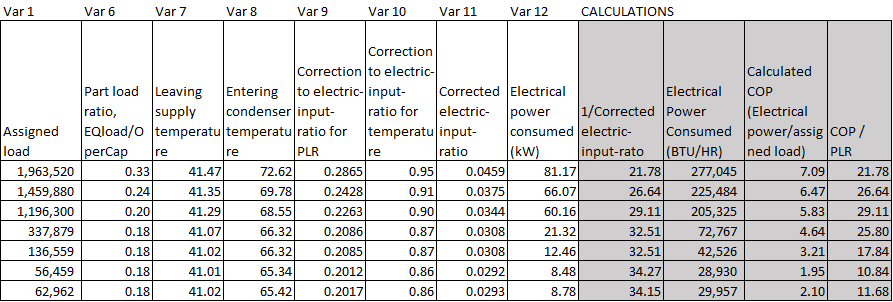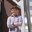Question-and-Answer Resource for the Building Energy Modeling Community
Get started with the Help page

# Corrected Electric-Input Ratio in eQuestWhat does the "corrected electric-input ratio" variable in eQuest represent? From what I can tell it isn't 1/COP, and it isn't the electric power divided by the rated capacity. In the image below, the data in white columns is from eQuest, and the grey columns are my calculations.edit retag close merge delete

Sort by » oldest newest most votedThis has to do with the specifics of the formula used by DOE2/eQUEST to calculate power. The "Corrected electric-input-ratio" is $$EIR\text{-}FT \times EIR\text{-}FPLR \times ELEC\text{-}INPUT\text{-}RATIO$$

where $ELEC\text{-}INPUT\text{-}RATIO$ is the nominal electric input ratio. The tricky thing is that the part load ratio is already accounted for in the $EIR\text{-}FPLR$ term. The formula for power is

$$CAPACITY\text{-}FT \times CAPACITY \times EIR\text{-}FT \times EIR\text{-}PLR \times ELEC\text{-}INPUT\text{-}RATIO / 3412$$

where $CAPACITY$ is the nominal capacity in Btu/hr. So the "Corrected electric-input-ratio" can also be calculated as the current power consumption (convert to Btu/hr) divided by the operating capacity, not as the power divided by the current load as you would think. Again, this just has to do with the way the formula works out and the fact that the $EIR\text{-}FPLR$ curve accounts for the part load ratio.

more

3

To elaborate on this answer, From your calculations: For the first three rows of data: The left most grey column (1/"corrected EIR") is equal to the right most grey column (COP/PLR). For the last four rows of data: the "Assigned Load" (left most white column) is less than the input value used for "Minimum Part Load Ratio" of the equipment. Therefore your system is cycling and running for a fraction of the one hour timestep at the minimum allowed part load ratio. Thus, you will need to factor out the fractional run time in order to see the equality observed for the first three rows of data.

How do I then find capacity? I would think it would be nominal capacity times PLR, but that doesn't seem right. And how do I find CAPACITY-FT since eQuest doesn't output it? Obviously if I knew how to calculate CAPACITY I would then be able to calculate this from the equation you provided above for power.

And Molly- that is a really useful observation. Thank you!

1

The $CAPACITY$ variable above is the rated capacity input parameter. The operating capacity is an output variable labeled "Operating capacity at current conditions". It accounts for a chiller having more or less ability to cool depending on evaporator/condenser temperatures. The operating capacity is the $CAPACITY\text{-}FT$ multiplied by the rated capacity; so, you can get $CAPACITY\text{-}FT$ by dividing the "Operating capacity at current conditions" by the rated capacity. You can also evaluate it directly by using the curve coefficients and the evaporator/condenser temperatures.

Thanks! Somehow I missed that CAPACITY is an output variable

Anna,

The formula eQuest uses is the following:

EER to EIR =(1/(EER)-0.012167)/((1/3.413)+0.012167) COP to HEIR =(1/(COP3.413)-0.012167)/(1/3.413 + 0.012167) HSPF to HEIR = (1/((HSPF0.28+1.13)*3.413)-0.012167)/((1/3.413)-0.012167)

This is an estimation the software makes in order to subtract the % of the EER that it assumes includes the fan power in the efficiency of just the condenser.

more